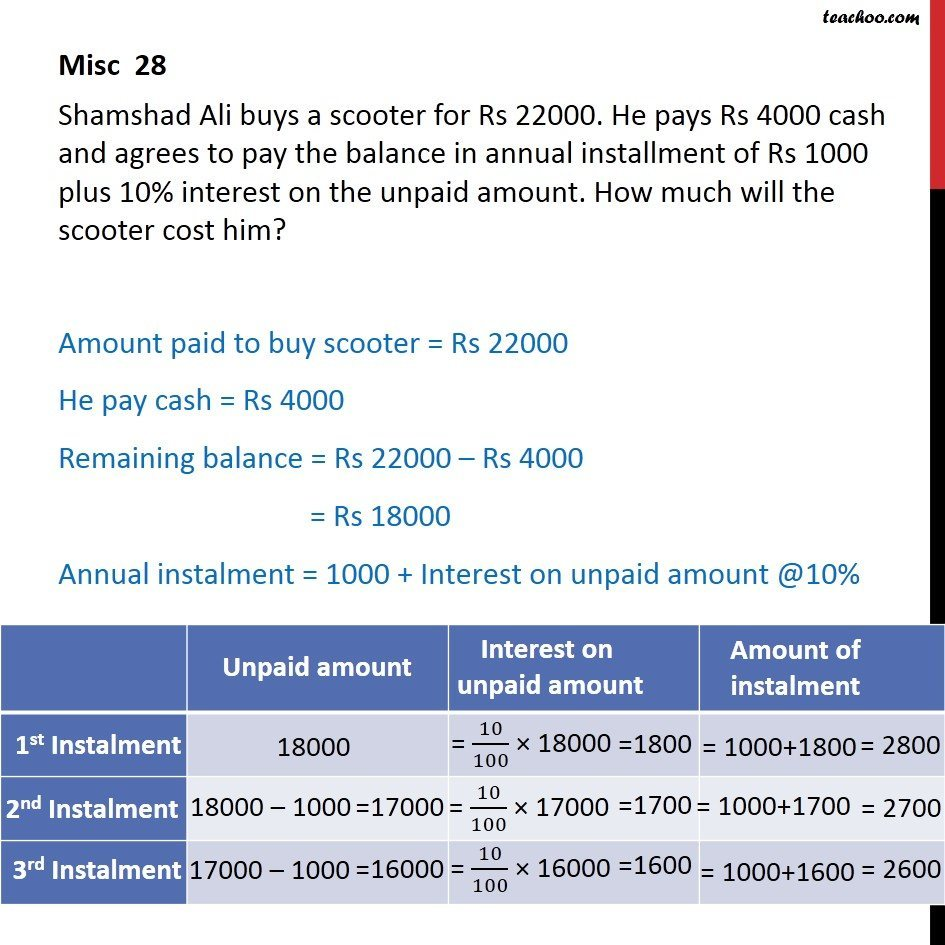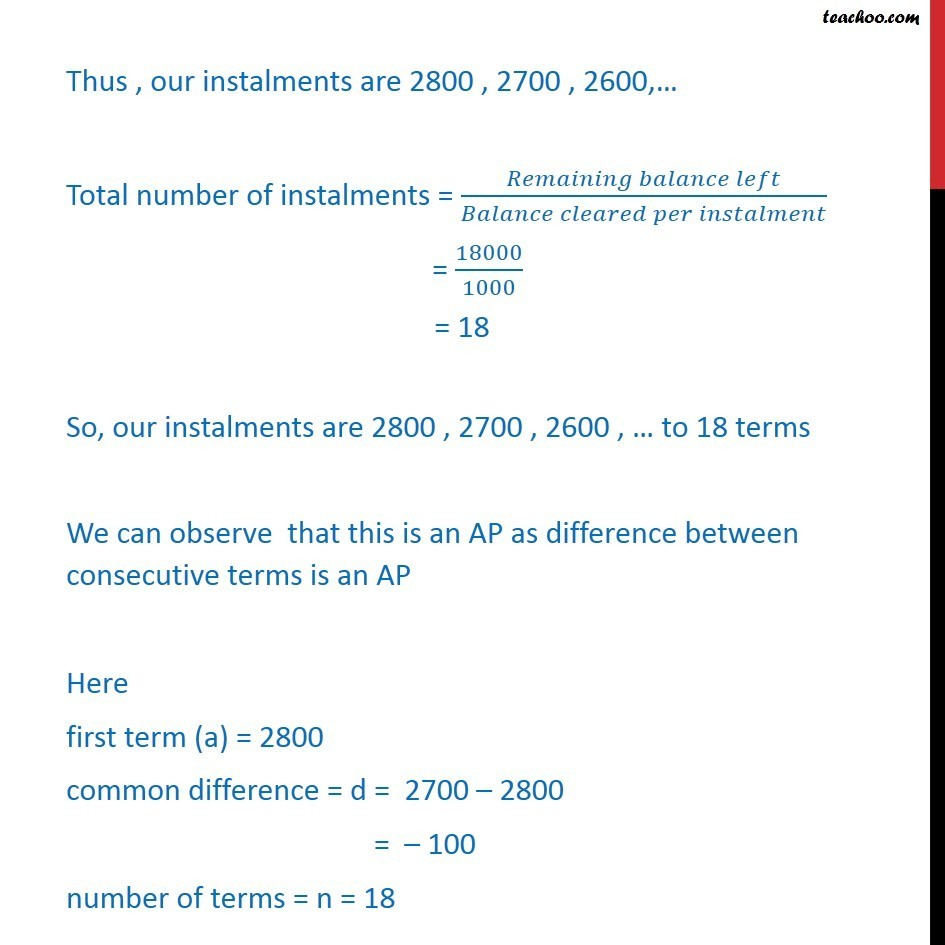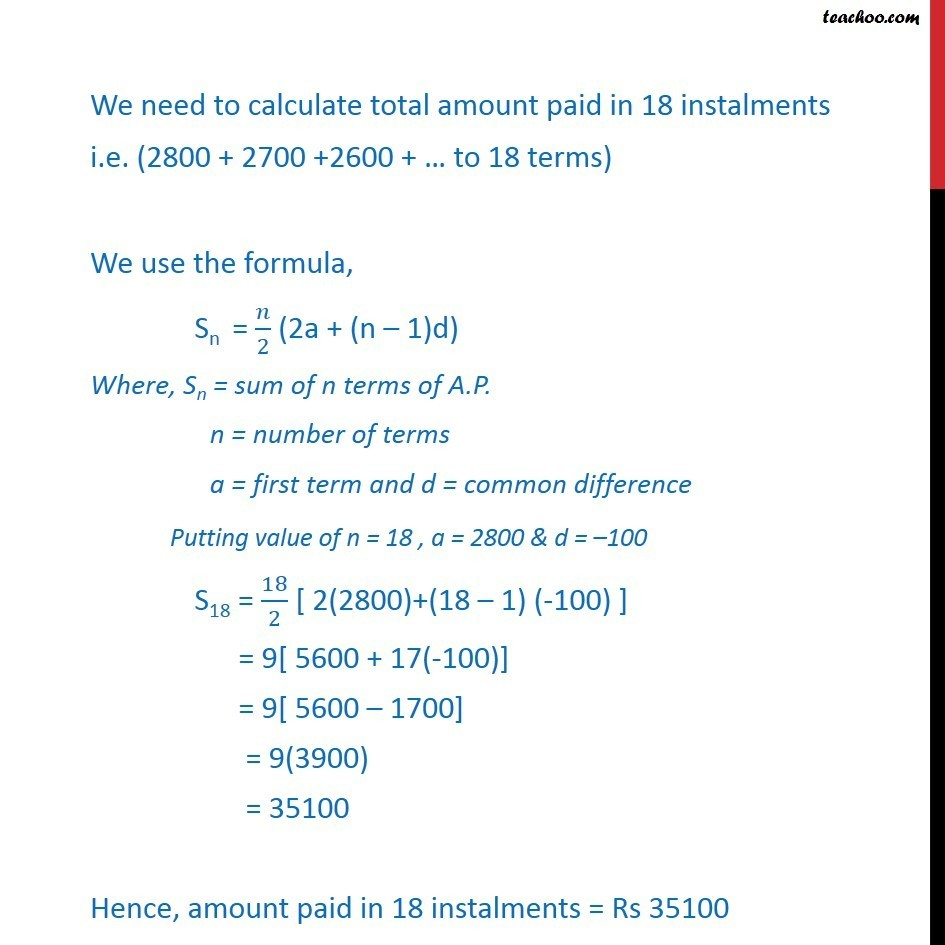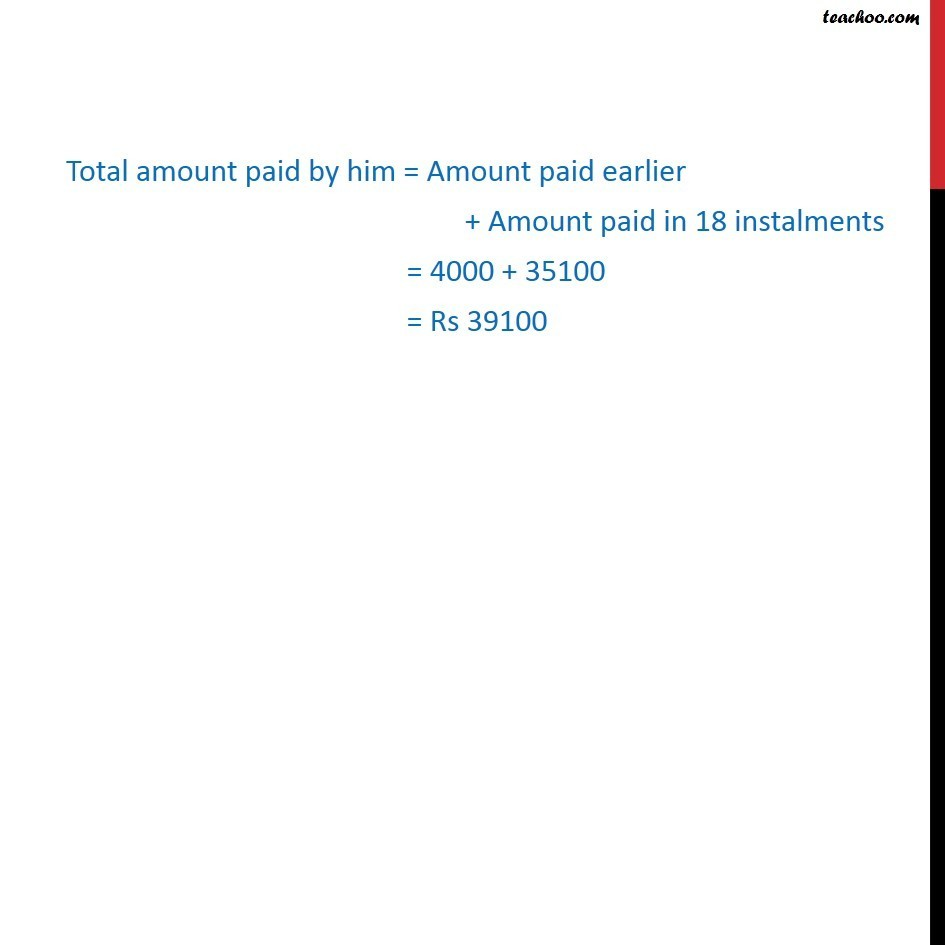Miscellaneous

Chapter 8 Class 11 Sequences and Series
Serial order wiseLearn in your speed, with individual attention - Teachoo Maths 1-on-1 Class

### Transcript

Misc 14 Shamshad Ali buys a scooter for Rs 22000. He pays Rs 4000 cash and agrees to pay the balance in annual installment of Rs 1000 plus 10% interest on the unpaid amount. How much will the scooter cost him? Amount paid to buy scooter = Rs 22000 He pay cash = Rs 4000 Remaining balance = Rs 22000 – Rs 4000 = Rs 18000 Annual instalment = 1000 + "Interest on unpaid amount @10%" Thus , our instalments are 2800 , 2700 , 2600,… Total number of instalments = (𝑅𝑒𝑚𝑎𝑖𝑛𝑖𝑛𝑔 𝑏𝑎𝑙𝑎𝑛𝑐𝑒 𝑙𝑒𝑓𝑡)/(𝐵𝑎𝑙𝑎𝑛𝑐𝑒 𝑐𝑙𝑒𝑎𝑟𝑒𝑑 𝑝𝑒𝑟 𝑖𝑛𝑠𝑡𝑎𝑙𝑚𝑒𝑛𝑡) = 18000/1000 = 18 So, our instalments are 2800 , 2700 , 2600 , … to 18 terms We can observe that this is an AP as difference between consecutive terms is an AP Here first term (a) = 2800 common difference = d = 2700 – 2800 = – 100 number of terms = n = 18 We need to calculate total amount paid in 18 instalments i.e. (2800 + 2700 +2600 + … to 18 terms) We use the formula, Sn = 𝑛/2 (2a + (n – 1)d) Where, Sn = sum of n terms of A.P. n = number of terms a = first term and d = common difference Putting value of n = 18 , a = 2800 & d = –100 S18 = 18/2 [ 2(2800)+(18 – 1) (-100) ] = 9[ 5600 + 17(-100)] = 9[ 5600 – 1700] = 9(3900) = 35100 Hence, amount paid in 18 instalments = Rs 35100 Total amount paid by him = Amount paid earlier + Amount paid in 18 instalments = 4000 + 35100 = Rs 39100